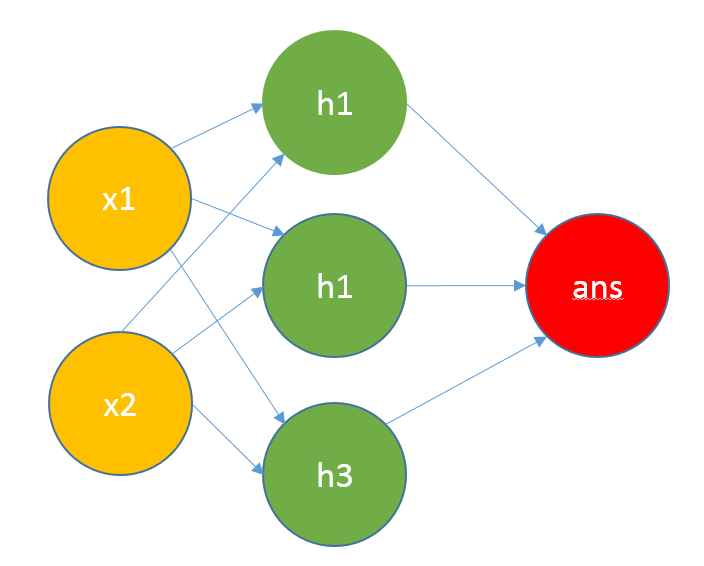# 函数的计算

## 一元函数

$f(x) = ax + b$

• 求值：一次性计算
• 求解：需要两组数值

## 多元函数

$f(x,y) = ax + by + c$

$F(x_1,...,x_n) = [x_1,...,x_n] \times \left[ \begin{matrix} w_1 \\ ... \\ w_n \end{matrix} \right] + c$

$F(X) = X \times W +C$

## 多组函数

$\left[\begin{matrix}x_{11}&x_{12}\\x_{21}&x_{22}\\ \end{matrix}\right] \times \left[\begin{matrix}w_{11}&w_{12}\\ w_{21}&w_{22}\\ \end{matrix}\right]= \left[\begin{matrix} x_{11} \times w_{11} + x_{12} \times w_{21} & x_{12} \times w_{12} + x_{12} \times w_{22} \\ x_{21} \times w_{11} + x_{22} \times w_{21} & x_{21} \times w_{12} + x_{22} \times w_{22} \\ \end{matrix}\right] \Rightarrow \left[\begin{matrix} a&b\\ c&d\end{matrix}\right]$

• 特征组

$\left[ \begin{matrix} w_{11}&w_{12}\\ w_{21}&w_{22} \end{matrix} \right]= \begin{cases} f_1(x, y) = x \times w_{11} + y \times w_{12} \\ f_2(x, y) = x \times w_{21} + y \times w_{22} \\ \end{cases}$

• 输入组

• 对照理解
1. 每个人有很多的特征
2. 每个特征散布很多人

• X：单样本多特征
• W：单映射多权重

• X：单特征多样本
• W：单权重多映射

# 特征的抽取

## 基本特征

$f(scorer) = \begin{cases} 及格&score \geq 60\\ 不及格&score\lt60 \end{cases}$

$f(x) = x \div radix \times100$

## 多种特征

$f_{heath}(high,weight) = \left| 1 - \frac{weight \times 10^4}{70 \times high - 5600} \right|$

$h_{test}(high, weight) = \begin{cases} 健康&f_{heath} \leq 10\\ 不健康&f_{heath} \gt 10 \end{cases}$

## 深度组合

• 健康（身高，体重）
• 学识（教育，思维）
• 呵护（物质，精神）# 计算的网络

## 多层网络# 自己的体会

• 特征提取

• 特征传递

• 基础属性覆盖完整
• 特征抽取没有偏差

## 重点的转移

$y = f(x)$

• 不用针对固定形式求解：因为基于线性组合传递，我们没有固定的表达式限制，不在意高次限制
• 问题求解的准确和普适：适用于处理任何的输入/输出问题，不关心内在处理

• 多少层
• 多少特征
• 参数几何